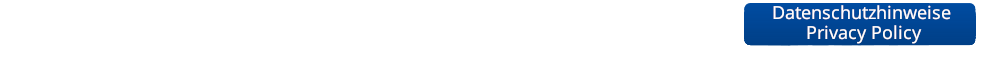Semester: SS 2019 Type: Lecture Lecturer: Credits: V3 + Ü1 (6 ECTS credits)
Course Dates:
Type
Date
Room
Lecture / Exercise Wednesday, 10:30 - 12:00 H06
Lecture / Exercise Thursday, 10:30 - 12:00 H04

Lecture Description

This lecture will extend the scope of the "Machine Learning" lecture with additional and, in parts, more advanced concepts. In particular, the lecture will cover the following areas:

• Regression techniques (linear regression, ridge regression, lasso, support vector regression)
• Probabilistic Graphical Models
• Exact inference
• Approximative Inference
• Learning with Latent Variables
• Deep Generative Models
• Deep Reinforcement Learning

Literature

We will mainly make use of the following books:

• C.M. Bishop, Pattern Recognition and Machine Learning, Springer, 2006
• I. Goodfellow, Y. Bengio, A. Courville, Deep Learning, 2016
• R.S. Sutton, A.G. Barto, Reinforcement Learning: An Introduction, 2nd Edition, MIT Press, 2018

However, a good part of the material presented in this class is the result of very recent research, so it hasn't found its way into textbooks yet. Wherever research papers are necessary for a deeper understanding, we will make them available on this web page.

Prerequisites

Successful completion of the class "Machine Learning" is recommended, but not a hard prerequisite.

Exercises

The class is accompanied by exercises that will allow you to collect hands-on experience with the algorithms introduced in the lecture. There will be both pen&paper exercises and practical programming exercises based on Matlab/numpy/TensorFlow (roughly 1 exercise sheet every 2 weeks). Please turn in your solutions to the exercises by e-mail to the appropriate TA the night before the exercise class.

We ask you to work in teams of 2-3 students.

Course Schedule
Date Title Content Material
Introduction Introduction, Polynomial Fitting, Least-Squares Regression, Overfitting, Regularization, Ridge Regression
Linear Regression I Probabilistic View of Regression, Maximum Likelihood, MAP, Bayesian Curve Fitting
Linear Regression II Basis Functions, Sequential Learning, Multiple Outputs, Regularization, Lasso, Bias-Variance Decomposition
Linear Regression III Kernels, Kernel Ridge Regression
Deep Reinforcement Learning I Reinforcement Learning, TD Learning, Q-Learning, SARSA, Deep RL
Deep Reinforcement Learning II Deep RL, Deep Q-Learning, Deep Policy Gradients, Case studies
Exercise 1 Regression, Least-Squares, Ridge, Kernel Ridge; Introduction to Tensorflow
Graphical Models I Intro to Graphical Models, Bayesian Networks, Conditional Independence, Bayes Ball algorithm
-- no class (May 1st)
Graphical Models II Markov Random Fields, Converting directed to undirected models, Factor Graphs
Graphical Models III Exact Inference, Message Passing, Belief Propagation, Factor Graphs, Sum-product algorithm, Max-sum algorithm
Graphical Models IV Junction Tree algorithm, Loopy BP, Applications of MRFs, CRFs
Graphical Models V Solving MRFs with Graph Cuts, s-t Mincut, Alpha Expansion
Exercise 2 Reinforment Learning in Tensorflow and OpenAI Gym
Approximate Inference I Sampling Approaches, Monte Carlo Methods, Transformation Sampling, Rejection Sampling, Importance Sampling
Approximate Inference II Markov Chain Monte Carlo, Metropolis-Hastings Algorithm, Gibbs Sampling
Latent Variable Models Latent Variable Models
- no class (Ascension)
Exercise 3 Bayes Nets, Inference, Belief Propagation
Latent Variable Models II Latent Variable view of EM, Generalized EM, Monte Carlo EM, Variational view of EM
- no class (Excursion week)
- no class (Excursion week)
Latent Variable Models III Bayesian Estimation Revisited, Bayesian Mixture Models, Approximate Inference for Bayesian Mixture Models
- no class (Corpus Christi)
GANs GANs
Exercise 4 Rejection Sampling, Importance Sampling, Metropolis-Hastings
VAEs VAEs
- no class
VAEs 2 VAEs 2
Repetition Repetition